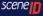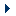# A.D.A. Amiga Demoscene Archive

 HOME DEMOS LOGOS PARTIES SCENERS CHARTS COMMENTS FORUM INFOAmiga Demoscene Archive Forum / Coding / Not so much a coding question as a maths question.

 Author Message #1 - Posted: 30 May 2007 23:52 Reply Quote OK. I have a line which goes from x1,y1 to x2,y2. If i'm standing at x1,y1 and looking at x2,y2, how can i tell if point x3,y3 is to my left or to my right? IE if i have to turn further to my right to be looking at x3,y3 than i would if i turned left, i consider x3,y3 to be on my left. #2 - Posted: 31 May 2007 01:07 Reply Quote Long answer: Define two vectors A = (x2-x1, y2-y1) B = (x3-x1, y3-y1) The angle, t, between them is given by the dot product: A dot B = |A||B|cos(t) , 0 <= t <= 180 degrees |A| > 0 and |B| > 0, so the sign of the dot product is equal to the sign of cos(t) which is negative when t > 90 degrees Now rotate A 90 degrees CCW: A' = (y1-y2, x2-x1) And if you sketch it out you'll see that any vector that makes a <90 degree angle with A' is to the left of the A vector, as seen along the length of A. So if A' dot B > 0, B is oriented CCW with respect to A if A' dot B = 0, your three points are collinear (not even a typo ;) if A' dot B < 0, B is oriented CW with respect to A (Switch the signs if your coordinate system has the y axis oriented downwards). I think that's easier to follow than the determinant/parallelogram/area explanation, but A' dot B is also the determinant of the 2x2 matrix made up by A and B as row vectors. Short answer: Check the sign of (y1-y2)*(x3-x1) + (x2-x1)*(y3-y1) #3 - Posted: 31 May 2007 01:09 - Edited Reply Quote I think i've got it. I think I need to calculate the dot product of the vector. So: dot_product = (x2-x1)*(x3-x1)+(y2-y1)*(y3-y1) If the dot product is positive, its on one side, and if negative its on the other. Right? Edit: Crap, you just posted that while i was posting this :) #4 - Posted: 31 May 2007 01:52 Reply Quote Well, you had it wrong, so hah! :) That'll tell you if (x3,y3) is in front of or behind you. Hence the 90 degree rotation. Anyway. I'm drawing donkeys. #5 - Posted: 31 May 2007 04:07 - Edited Reply Quote Note: the post below contains inebriated ramblings which subsequently have been proven to have no basis in reality. ```dot_product = (x2-x1)*(x3-x1)+(y2-y1)*(y3-y1) If the dot product is positive, its on one side, and if negative its on the other. Right?``` If you're just pulling the formula out of your behind, then that is not a dot product. It has a pattern which is different from, but similar to, the following: 1) the Z component of a cross product of vector 1 & vector 2 2) twice the signed area of the triangle formed by (x1,y1), (x2,y2), (x3,y3) (this is what doom is getting at, albeit in an abridged version) Both of those can be used to detemine whether the triangle formed by the (x1,y1), (x2,y2), (x3,y3) vertices are ordered in a clockwise or counterclockwise fashion. And that test can be used to answer whether or not (x3,y3) are "to the left of" (x1,y1) .. (x2,y2). #6 - Posted: 31 May 2007 10:18 - Edited Reply Quote @Kalms Oh. Well, i figured it out after reading this: http://www.ucl.ac.uk/Mathematics/geomath/vecsnb/MH vec.html (section entitled "Multiplying vectors I: the scalar (or dot) product") so i didn't exactly pull it out of my behind. #7 - Posted: 31 May 2007 10:21 Reply Quote @Doom Yep. You are right and I am wrong! #8 - Posted: 31 May 2007 17:29 Reply Quote Kalms is wrong too tough (or cryptic?). (x2-x1)*(x3-x1)+(y2-y1)*(y3-y1) is the dot product of (x2-x1, y2-y1) and (x3-x1, y3-y1), and those are the two relevant vectors. If he's referring to my (y1-y2)*(x3-x1)+(x2-x1)*(y3-y1), then that is a dot product too, just not of the same too vectors. Nothing incorrect about the maths, and I really do believe it's easier to just rotate one vector in the explanation rather than add a dimension and look at the direction of the cross product, work with signed areas (not very intuitive), or some of the other approaches that arrive at the exact same formula anyway. #9 - Posted: 31 May 2007 18:34 Reply Quote @doom Well, I understood exactly what you meant in your post, and I understood how you came to your equation, and what was wrong with me just trying to use a dot product of the two vectors, so I think you explained it quite well. #10 - Posted: 31 May 2007 18:37 Reply Quote Er. I'm not exactly sure how I thought when I wrote that forum post. Perhaps I should avoid posting after doing a pub-round in the future? Suffice to say that I'm wrong and doom is right. :) #11 - Posted: 31 May 2007 21:11 Reply Quote A bit off-topic but how important is the understanding of vectors for coding? In which areas/effects are vectors important. It would be nice to have a general idea on this, just out of interest. (i've had my fair share of maths when i was studying, but having never used any of it after school, sadly, it's all gone... and i mean ALL :( ... i didn't hate maths but it was not my favourite subject either.) #12 - Posted: 31 May 2007 22:15 Reply Quote If you want to do something 3D graphics related, it is extremely useful. You *can* get by without knowing a lot of vector maths (linear algebra). However, Linear algebra gives you a well-defined set of tools, which are suited for the purpose. Thinking and writing formulas using vector notation is more concise than using plain high school maths. If you solve a problem with vector maths in 1d or 2d, you can often generalize it to 3d in a few simple steps. After a while, you will learn what your tools are (mainly vectors, lines, planes and transformation matrices) and how they can be used. Any problem you encounter, you learn to decompose into a set of operations using these primitives. #13 - Posted: 1 Jun 2007 00:53 Reply Quote Hah. Back from the pub. Better post something now. But Kalms said it all. :) Except I'd add a suggestion that anyone who's serious about coding 3D effects get a textbook on linear algebra. And/or, and this may or may not be even better, go here for some pretty good video lectures on the subject (sadly in RealMedia format, get MPC and Real Alternative). Some understanding of vectors and calculus is required to follow all of it, IIRC.